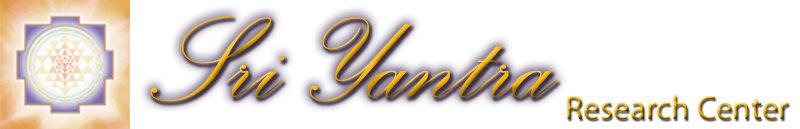How to Evaluate a Sri Yantra In a previous article we have explained that three criteria are necessary for an optimal Sri Yantra configuration. The following article will explain how you can test any Sri Yantra using a ruler and compass or a drawing program such as Visio to find out how close or far a specific configuration is from the ideal optimal configuration. The first and probably most obvious criterion is concurrency or the degree of precision at the triple intersections. The second is concentricity which requires the center of the innermost triangle (bindu location) to be also at the center of the outer circle. The third is equilaterality, where the innermost triangle should be perfectly symmetrical (equilateral triangle). 1. Concurrency (Precision)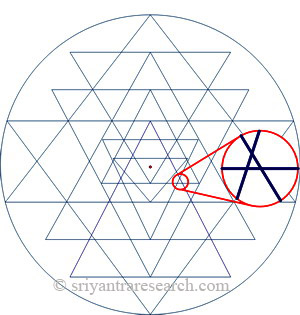Figure 1. Error at a triple intersection This criteria is the most obvious. The triple intersections must intersect precisely at one point as oppose to forming secondary triangles. First one should look at how many errors are present in the figure. If the figure contains more than two errors then this indicates that the figure was not drawn properly. When following the logical order shown here a maximum of two errors will be found. The other question is how big the errors are. ideally the errors are so small that they are hidden by the thickness of the lines used. A mathematical value can be given for the total errors by adding all the area of the errors and relating this number to the area of the circle as a percentage. (nb errors x error surface / circle area) x 100 2. Concentricity (Centeredness)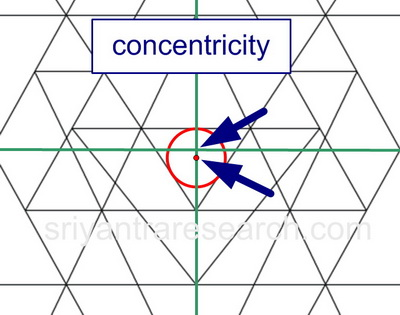Figure 2. Example of a non-concentric Sri Yantra. First you need to find the center of the outer triangle. This can be done easily in a drawing program or with a compass. In the drawing above the center of the outer circle is shown with the green lines. Now we need of find the proper location of the bindu. In many figures the bindu is not located properly, i.e. at the center of the innermost triangle. The simplest way to find the correct location of the bind is to draw a circle that fits perfectly inside the innermost triangle. This is called the incenter of a triangle. Ideally the bindu should be at the center of the innermost triangle AND at centered of the outer circle. The figure above shows an example of a figure where the center of the innermost triangle (bindu) does not coincide with the center of the outer cirlce (green lines). This can be expressed mathematicaly by calculating: (distance between bindu and circle center / circle diameter ) * 100 3. Equilaterality (Symmetry)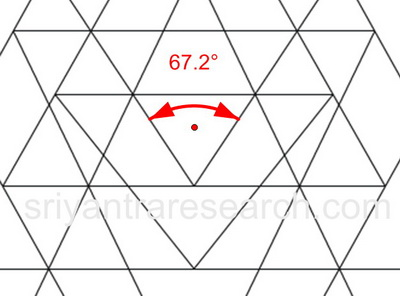Figure 3. Equilaterality Measure the angle of the innermost triangle. The innermost triangle should be equilateral for reasons explained here. In the figure above we see a figure where the innermost triangle is not equilateral since the angle is different from 60 degrees. An equilateral triangle is symmetrical because all sides are the same lenght and all angles are equal to 60 degrees.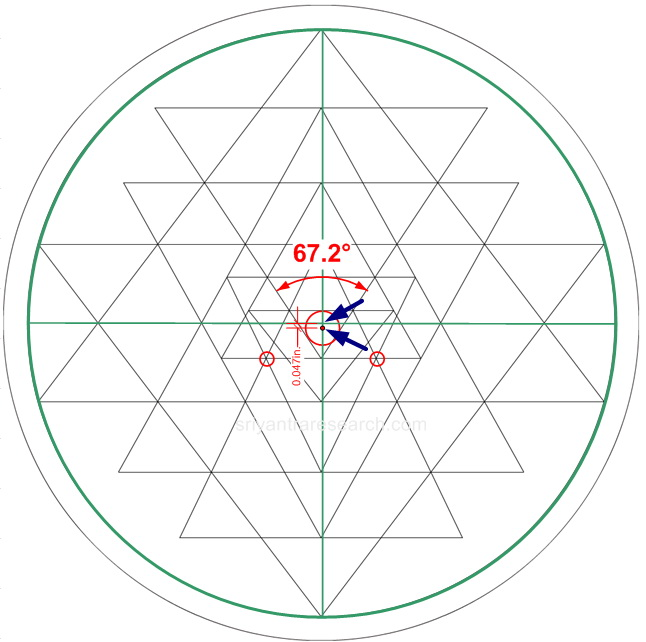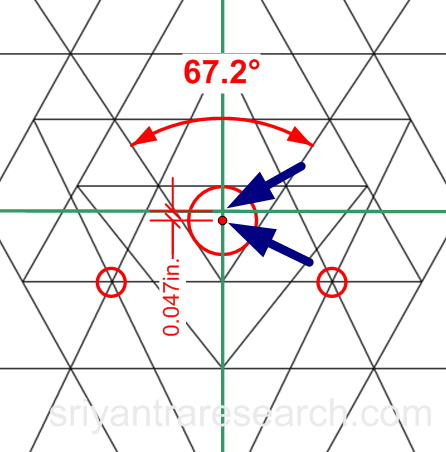We can see that the figure above used as an example doesn't satisfy all three criteria. The first criterion of concurrency is satisfied since all the intersection match precisely. However the center of the innmermost triangle (bindu location) is not at the center of the outer circle (green cross). Also we see that the angle of the innermost triangle is 67.2 degrees, or 7.2 degree away from the ideal of 60 degrees.